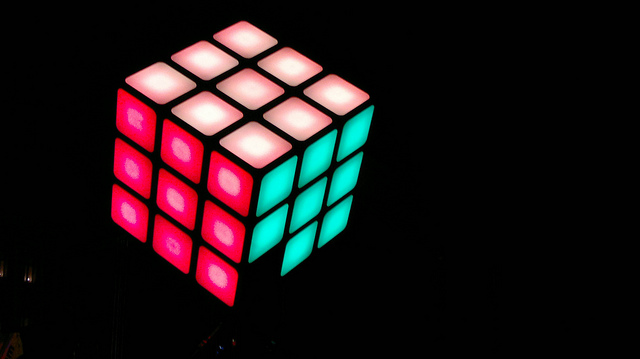# BIg Rubik's Cube

Probability Level 2There are 8 cubes in a $2^{3}$ Rubik's Cube or Pocket Cube and there are 26 cubes in a $3^{3}$ Rubik's Cube. K is the number of cubes in a $2511^{3}$ Rubik's Cube. What are the last 3 digits of K?

×